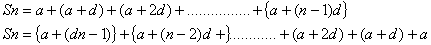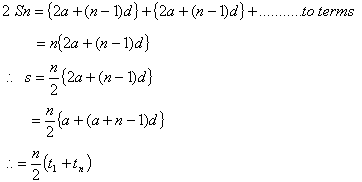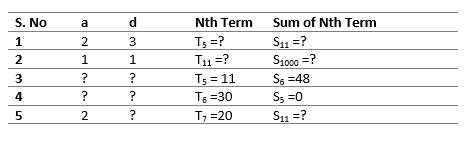# Arithmetic Progressions Class 10 Notes

In this page we will explain the topics for the chapter 5 of Arithmetic Progressions Class 10 Maths.We have given quality Arithmetic Progressions Class 10 Notes to explain various things so that students can benefits from it and learn maths in a fun and easy manner, Hope you like them and do not forget to like , social share and comment at the end of the page.
Table of Content

## Introduction:

• We see lot of pattern in our daily lives.  For example, Rohan salary in his first year is 2000 and he will be getting increment of 500 every year,So his salary for First, second, third, fourth …. Will be of the form 2000,2500,3000,3500
• So here we see the pattern in Rohan salary. The difference between two consecutive year salaries is constant. In the similar, world around us present us these pattern in many form. In this chapter, we will learn a special type of pattern called Arithmetic Progression

## What is arithmetic progression?

An arithmetic progression is a sequence of numbers such that the difference of any two successive members is a constant
Let us consider following series
(1)$1,5,9,13,17....$
(2)$1,2,3,4,5,...$
(3)$7,7,7,7.....$
All these sets follow certain rules. In first set $5 - 1 = 9 - 5 = 13 - 9 = 17 - 13 = 4$
In second set $2 - 1 = 3 - 2 = 4 - 3 = 1$
and so on.
Here the difference between any successive members is a constant
Such series are called Arithmetic Progression

## Some Important points about AP

1. The difference is called the common difference of the AP and It is denoted by d
2. The members are called terms. The first member is called first term
3. We can denote common difference  by d
4. If $a_1, a_2,a_3,a_4,a_5$ are the terms in AP then
$D=a_2 - a_1 =a_3 - a_2 =a_4 - a_3=a_5 - a_4$
5. We can represent the general form of AP in the form
$a,a+d,a+2d,a+3d,a+4d,.....$
Where $a$ is first term and $d$ is the common difference
6. If the AP series has last term then it s finite Arithmetic Progression and if the AP series has infinite then it is called the Inifinite Arithmetic Progression

Example: Find if the below series is Arithmetic Progression
$1, 4, 7, 10, 13, 16, 19, 22, 25,...$
Solution:
$D=3=3=3=3$
So it is AP
Practice Questions
Observe the number sequence and complete the next two terms of the sequence
• $2, 8, 14, 20$
• $5, 13, 21, 29$
• $11, 22, 33, 44$
• $-3, 1,5, 9$
• $5, -1, -7, -13$

## nth term of Arithmetic Progression

On the basis of above discussion we can consider the following series
$a, a + d, a + 2d, a + 3d, .........................$
Here $a = 1$, $d = 4$
$a + d = 1 + 4 = 5$
$a + 2d = 1 + 2 x 4 = 9$
and so on
Thus we can say that
$a$ = First term
$a + d$ = Second term
$a + 2d$ = Third term
$a + 3d$ = Fourth term and son on
$n_{th} \; term = a + (n - 1)d$
Here First term = $t_1= a$
Second term = $t_2 = a + d$
and hence, $t_n= a + (n - 1)d$
d is called common difference and the series is called arithmetic progression
Example 1
Find the 13th term of the AP : 1, 5, 9, . . .
Solution
Here, $a = 1, d = 5 - 1 = 4 \; and \; n = 13$
We have $a_n = a + (n - 1) d$
So, $a_{13} = 1 + (13 - 1) \times 5 = 1 + 60 = 61$
Therefore, the 13th term of the given AP is 61.

Example 2
Which term of the AP : 21, 18, 15, . . . is -81? Also, is any term 0? Give reason for your answer.
Solution
Here, $a = 21, d = 18 - 21 = - 3 \; and \; a_n = -81$, and we have to find n.
As $a_n = a + (n - 1) d$
we have $-81 = 21 + (n - 1)( -3)$
$- 81 = 24 - 3n$
$-105 = -3n$
So, n = 35
Therefore, the 35th term of the given AP is -81.
Next, we want to know if there is any n for which $a_n = 0$. If such an n is there, then
$21 + (n - 1) (-3) = 0$
i.e., $3(n - 1) = 21$
i.e., n = 8
So, the eighth term is 0.
Example 3
Determine the AP whose 3rd term is 5 and the 7th term is 9.
Solution
We have
$a_3 = a + (3 - 1) d = a + 2d = 5$ (1)
and $a_7 = a + (7 - 1) d = a + 6d = 9$ (2)
Solving the pair of linear equations (1) and (2), we get
a = 3, d = 1
Hence, the required AP is 3, 4, 5, 6, 7, . . .

## Sum of n items in Arithmetic Progression

Let $S = 1 + 2 + 3 + 4 + 5 + 6 + 7 + + 8 + 9 + 10$ and writting in reversed order
$S = 10 + 9 + 8 + 7 + 6 + 5 + 4 + 3 + 2 + 1$
Adding these two we get $2s = 11 + 11 + 11 + 11 + 11 + 11 + 11 = 11 + 11 + 11$
$2S= 10 \times 11$
$S = \frac {(10 \times 11)}{2} = 55$
In similar way, ifExample
Find the sum of first 24 terms of the list of numbers whose nth term is given by
$a_n = 5 + 2n$
Solution
As $a_n = 5 + 2n$
so, $a_1 = 5 + 2 = 7$
$a_2 = 5 + 2 \times 2 = 9$
$a_3 = 5 + 2 \times 3 = 11$
So,
List of numbers becomes 7, 9, 11, . . .
Here, 9 - 7 = 11 - 9 = 2 and so on.
So, it forms an AP with common difference d = 2.
To find $S_{24}$, we have n = 24, a = 7, d = 2.
Therefore, $S_{24} = \frac {24}{2}[2 \times 7+(24 -1) 2]$ = 12 [14 + 46] = 720
So, sum of first 24 terms of the list of numbers is 720

## Arithmetic Mean

If a,b,c are in AP, then $b=\frac {(a+c)}{2}$ and b is called the Arithmetic Mean

## Practice Questions### Quiz Time

Question 1 If the first term of an AP is -5 and the common difference is 2, then the sum of the first 6 terms is
A) 15
B) 5
C) 0
D) 11
Question 2 If the common difference of an AP is 6, then what is $a_{18} -a_{13}$ ? A) 36
B) 30
C) 42
D) None of the above
Question 3 The sum of first n positive integers is given by
A) $\frac {n(n+1)}{2}$
B) $\frac {n(n-1)}{2}$
C) $\frac {n(n+1)}{4}$
D) $\frac {n(n+1)}{3}$
Question 4 which of following is not an AP
A) 1,3,5,7...
B) -15,10,-5,0,5
C) $2 + \sqrt {2}, 2+ \sqrt {8},2+\sqrt {18},...$
D) -1,1,3,6......
Question 5 In an AP if $a = -7.2, d = 3.6, a_n = 7.2$, then n is?
A) 1
B) 5
C)3
D)4
Question 6The arithmetic Means of 12 and 14 is
A) 13
B) 15
C) 16
D) 11

## Summary

Here is the Arithmetic Progressions Class 10 Maths Notes Summary
• We can represent the general form of AP in the form
$a,a+d,a+2d,a+3d,a+4d,.....$
Where $a$ is first term and $d$ is the common difference
• nth term is given by $a_n =a + (n-1)d$
• Sum of n term $S_n= \frac {n}{2} [2a + (n-1)d]$
• Arithmetic mean is give by $A= \frac {a+b}{2}$Go back to Class 10 Main Page using below links

### Practice Question

Question 1 What is $1 - \sqrt {3}$ ?
A) Non terminating repeating
B) Non terminating non repeating
C) Terminating
D) None of the above
Question 2 The volume of the largest right circular cone that can be cut out from a cube of edge 4.2 cm is?
A) 19.4 cm3
B) 12 cm3
C) 78.6 cm3
D) 58.2 cm3
Question 3 The sum of the first three terms of an AP is 33. If the product of the first and the third term exceeds the second term by 29, the AP is ?
A) 2 ,21,11
B) 1,10,19
C) -1 ,8,17
D) 2 ,11,20